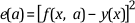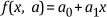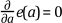# Least Squares Method

## LabVIEW 2018 Help

Edition Date: March 2018
Part Number: 371361R-01
View Product InfoLabVIEW 2016 HelpLabVIEW 2017 HelpLabVIEW 2018 HelpLabVIEW 2019 HelpLabVIEW 2020 Help

The least squares method of curve fitting fits a curve to a particular data set. The following equation defines the least square error.where e(a) is the least square error, y(x) is the observed data set, f(x, a) is the functional description of the data set, and a is the set of curve coefficients that best describes the curve.

For example, if a = {a0, a1}, the following equation yields the functional description:The least squares algorithm finds a by solving the system defined by the following equation.To solve the system defined by the previous equation, you set up and solve the Jacobian system generated by expanding the previous equation. After you solve the system for a, you can use the functional description f(x, a) to obtain an estimate of the observed data set for any value of x.